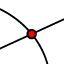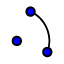# Circles

Topic:
Circle

## Step 1

Use the LINE toolto construct a line through Center A and Point B.

## Step 2

Construct a Point C on the circle using the POINT tool.

## Step 3

Use the LINEtool to construct a line through Center A and Point C.

## Step 4

Click the Point tool in the toolbar. Then use the INTERSECTION﻿tool to construct Point D and Point E. (Point D should intersect line AB and the circle. Point E should intersect line AC and the circle.)

## Step 5

Use the ANGLE MEASUREtool to create and measure angle BAC and angle DAE. *First select the point B, then center A, then select point C counterclockwise!* *First select the point D, then center A, then select point E counterclockwise!*

## Step 6

Use the CIRCULAR ARCtool to create and measure Arc BC and Arc DE. *First select the center A, then select Points B and C counterclockwise!* *First select the center A, then select Points D and E counterclockwise!*

## Step 7

Use the Algebra window to see the measures of Arc BC (labeled "d") and Arc DE (labeled "e").

## Step 8

Use the SEGMENTtool to construct Chord "h" with endpoints B and C. Use the SEGMENTtool to construct Chord "i" with endpoints D and E.

## Step 9

Use the Algebra Window to see the measures of Chord h and Chord i.